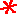Main      Site Guide### Scrambled Equations

• Scrambled Equations

In each of these puzzles, you are given a number that you must construct out of several other numbers. You do this by taking the numbers and performing addition, subtraction, multiplication, and/or division operations on them. Each number must be used in the calculations exactly once, and only these four operations listed may be used. You may parenthesize your expression however you wish. For example, 5 may be obtained from 1, 2, and 3, with the expression (3 + 2) × 1.

In many if not most cases, multiple solutions are possible, but usually only one is given on the solution page.

## #26

Make 9 out of 5, 6, 8, and 8.

Hint

Solution

## #27

Make 66 out of 2, 4, 4, and 8.

Hint

Solution

## #28

Make 54 out of 4, 6, 6, and 9.

Hint

Solution

## #29

Make 1 out of 1, 5, 6, and 8.

Hint

Solution

## #30

Make 32 out of 4, 7, 7, and 9.

Hint

Solution

## #31

Make 64 out of 2, 3, 4, and 6.

Hint

Solution

## #32Make 48 out of 1, 6, 8, and 9.

Hint

Solution

## #33

Make 12 out of 1, 4, 7, and 7.

Hint

Solution

## #34

Make 27 out of 1, 4, 7, and 7.

Hint

Solution

## #35

Make 50 out of 4, 6, 6, and 9.

Hint

Solution

## #36

Make 2 out of 1, 5, 7, and 7.

Hint

Solution

## #37

Make 19 out of 1, 4, 5, and 7.

Hint

Solution

## #38

Make 14 out of 1, 3, 5, and 8.

Hint

Solution

## #39

Make 34 out of 1, 7, 7, and 8.

Hint

Solution

## #40Make 44 out of 6, 7, 8, and 9.

Hint

Solution

## #41

Make 11 out of 7, 7, 9, and 9.

Hint

Solution

## #42

Make 22 out of 5, 6, 9, and 9.

Hint

Solution

## #43

Make 30 out of 2, 2, 5, and 7.

Hint

Solution

## #44Make 14 out of 2, 2, 5, and 7.

Hint

Solution

## #45

Make 38 out of 2, 3, 6, and 7.

Hint

Solution

## #46

Make 20 out of 3, 5, 7, and 9.

Hint

Solution

## #47

Make 44 out of 4, 7, 8, and 9.

Hint

Solution

## #48

Make 21 out of 1, 3, 8, and 8.

Hint

Solution

## #49

Make 45 out of 2, 5, 6, and 9.

Hint

Solution

## #50

Make 24 out of 1, 4, 5, and 6.

Hint

Solution